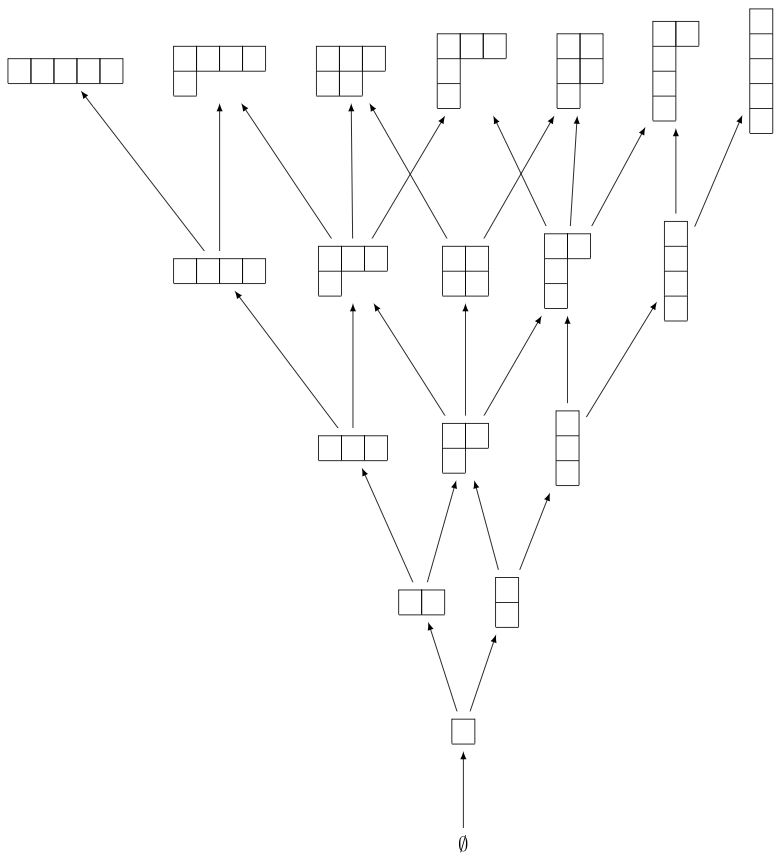# Young’s lattice and the RSK algorithm¶

This section provides some examples on Young’s lattice and the RSK (Robinson-Schensted-Knuth) algorithm explained in Chapter 8 of Stanley’s book [Stanley2013].

## Young’s Lattice¶

We begin by creating the first few levels of Young’s lattice $$Y$$. For this, we need to define the elements and the order relation for the poset, which is containment of partitions:

sage: level = 6
sage: elements = [b for n in range(level) for b in Partitions(n)]
sage: ord = lambda x,y: y.contains(x)
sage: H = Y.hasse_diagram()
sage: view(H)  # optional - dot2tex graphvizWe can now define the up and down operators $$U$$ and $$D$$ on $$\QQ Y$$. First we do so on partitions, which form a basis for $$\QQ Y$$:

sage: QQY = CombinatorialFreeModule(QQ,elements)

sage: def U_on_basis(la):
....:     covers = Y.upper_covers(la)
....:     return QQY.sum_of_monomials(covers)

sage: def D_on_basis(la):
....:     covers = Y.lower_covers(la)
....:     return QQY.sum_of_monomials(covers)


As a shorthand, one also can write the above as:

sage: U_on_basis = QQY.sum_of_monomials * Y.upper_covers
sage: D_on_basis = QQY.sum_of_monomials * Y.lower_covers


Here is the result when we apply the operators to the partition $$(2,1)$$:

sage: la = Partition([2,1])
sage: U_on_basis(la)
B[[2, 1, 1]] + B[[2, 2]] + B[[3, 1]]
sage: D_on_basis(la)
B[[1, 1]] + B[]


Now we define the up and down operator on $$\QQ Y$$:

sage: U = QQY.module_morphism(U_on_basis)
sage: D = QQY.module_morphism(D_on_basis)


We can check the identity $$D_{i+1} U_i - U_{i-1} D_i = I_i$$ explicitly on all partitions of $$i=3$$:

sage: for p in Partitions(3):
....:     b = QQY(p)
....:     assert D(U(b)) - U(D(b)) == b


We can also check that the coefficient of $$\lambda \vdash n$$ in $$U^n(\emptyset)$$ is equal to the number of standard Young tableaux of shape $$\lambda$$:

sage: u = QQY(Partition([]))
sage: for i in range(4):
....:     u = U(u)
sage: u
B[[1, 1, 1, 1]] + 3*B[[2, 1, 1]] + 2*B[[2, 2]] + 3*B[[3, 1]] + B[]


For example, the number of standard Young tableaux of shape $$(2,1,1)$$ is $$3$$:

sage: StandardTableaux([2,1,1]).cardinality()
3


We can test this in general:

sage: for la in u.support():
....:     assert u[la] == StandardTableaux(la).cardinality()


We can also check this against the hook length formula (Theorem 8.1):

sage: def hook_length_formula(p):
....:     n = p.size()
....:     return factorial(n) // prod(p.hook_length(*c) for c in p.cells())

sage: for la in u.support():
....:     assert u[la] == hook_length_formula(la)


## RSK Algorithm¶

Let us now turn to the RSK algorithm. We can verify Example 8.12 as follows:

sage: p = Permutation([4,2,7,3,6,1,5])
sage: RSK(p)
[[[1, 3, 5], [2, 6], [4, 7]], [[1, 3, 5], [2, 4], [6, 7]]]


The tableaux can also be displayed as tableaux:

sage: P,Q = RSK(p)
sage: P.pp()
1  3  5
2  6
4  7
sage: Q.pp()
1  3  5
2  4
6  7


The inverse RSK algorithm is implemented as follows:

sage: RSK_inverse(P,Q, output='permutation')
[4, 2, 7, 3, 6, 1, 5]


We can verify that the RSK algorithm is a bijection:

sage: def check_RSK(n):
....:     for p in Permutations(n):
....:          assert RSK_inverse(*RSK(p), output='permutation') == p
sage: for n in range(5):
....:     check_RSK(n)